# Length - math word problems

1. Squares ratioThe first square has a side length of a = 6 cm. The second square has a circumference of 6 dm. Calculate the proportions of the perimeters and the proportions of the contents of these squares? (Write the ratio in the basic form). (Perimeter = 4 * a, conte
2. What percentageWhat percentage of the Earth’s surface is seen by an astronaut from a height of h = 350 km. Take the Earth as a sphere with the radius R = 6370 km
3. Self-oscillation period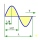The water in the vessel carried by the boy has a self-oscillation period of 0.8 s. What is the size of the boy's movement speed when the length of the boy's step is 60 cm? Give the result in m/s.
4. Steps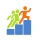Find the height between the two floors if you know that the number of steps between the two floors is 18, the gradient is 30º and the length of the step is 28.6 cm. Report the result in centimeters to the nearest centimeter.
5. Marlon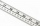Marlon drew a scale drawing of a summer camp. In real life, the sand volleyball court is 8 meters wide. It is 4 centimeters wide in the drawing. What is the drawing's scale factor? Simplify your answer and write it as a ratio, using a colon.
6. Altitude difference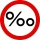What a climb in per mille of the hill long 4 km and the altitude difference is 6 meters?
7. Hiking trail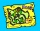The newly built hiking trail leads 25% through the field, 3/8 of the trail leads through the forest and the remaining 9 km along the river. How long is the train?
8. SupposeSuppose you know that the length of a line segment is 15, x2=6, y2=14 and x1= -3. Find the possible value of y1. Is there more than one possible answer? Why or why not?
9. A cell tower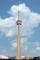A cell tower is located at coordinates (-5, -7) and has a circular range of 12 units. If Mr. XYZ is located at coordinates (4,5), will he be able to get a signal?
10. Triangular pyramidA regular tetrahedron is a triangular pyramid whose base and walls are identical equilateral triangles. Calculate the height of this body if the edge length is a = 8 cm
11. Two chordsIn a circle with radius r = 26 cm two parallel chords are drawn. One chord has a length t1 = 48 cm and the second has a length t2 = 20 cm, with the center lying between them. Calculate the distance of two chords.The quadrilateral pyramid has a rectangular base of 24 cm x 3.2dm and a body height of 0.4m. Calculate its volume and surface area.
13. Rotaty motionWhat is the minimum speed and frequency that we need to rotate with water can in a vertical plane along a circle with a radius of 70 cm to prevent water from spilling?
14. Newtonmeters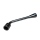The driver loosened the nut on the car wheel with a wrench that held 20 cm from the axis of the bolt. He acted on the key with a force of 320N. At what moment did he act on the bolt?
15. A drone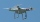A flying drone aimed the area for an architect. He took off perpendicularly from point C to point D. He was at a height of 300 m above the plane of ABC. The drone from point D pointed at a BDC angle of 43°. Calculate the distance between points C and B in
16. Two trains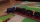Two trains departed from City A and City B against each other. They met after some time. The first train then took 9 hours to reach city B, and the second train took 4 hours to reach city A. In what proportion were the train speeds?
17. Cylinder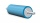The 1.8m cylinder contains 2000 liters of water. What area (in dm2) of this container is the water?
18. Parametric formCalculate the distance of point A [2,1] from the line p: X = -1 + 3 t Y = 5-4 t Line p has a parametric form of the line equation. ..
19. Possible lengths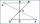Find the most possible lengths for the third side of a triangle with sides 20 and 18.
20. Doug bikedDoug biked 5 1/4 miles in 3/4 of an hour. What is his average speed?

Do you have an interesting mathematical word problem that you can't solve it? Submit math problem, and we can try to solve it.

We will send a solution to your e-mail address. Solved examples are also published here. Please enter the e-mail correctly and check whether you don't have a full mailbox.

Please do not submit problems from current active competitions such as Mathematical Olympiad, correspondence seminars etc...

Do you want to convert length units?